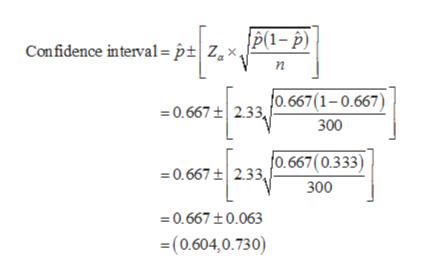# The Fox TV network is considering replacing one of its prime-time crime investigation shows with a new family-oriented comedy show. Before a final decision is made, network executives commission a sample of 300 viewers. After viewing the shows, 200 indicated they would watch the new show and suggested it replace the crime investigation show.a) Estimate the value of the population proportion. (Round your answers to 3 decimal places.)b) Develop a 98% confidence interval for the population proportion. (Use  z Distribution Table.) (Round your answers to 3 decimal places.)

Question

The Fox TV network is considering replacing one of its prime-time crime investigation shows with a new family-oriented comedy show. Before a final decision is made, network executives commission a sample of 300 viewers. After viewing the shows, 200 indicated they would watch the new show and suggested it replace the crime investigation show.

a) Estimate the value of the population proportion. (Round your answers to 3 decimal places.)

b) Develop a 98% confidence interval for the population proportion. (Use  z Distribution Table.) (Round your answers to 3 decimal places.)

check_circleExpert Solution
Step 1

a.The value of the population proportion is estimated below:

From the given information, in a random sample 300 persons 200 responded that they will watch the new show and suggested it replace the crime investigation show.

The sample proportion is unbiased estimator of population proportion.

The required population proportion is,

Step 2

Obtain the critical value at 98% confidence level:

The level of significance=(1–0.98)/2=0.01.

The required confidence level from left tail normal table=0.98+0.01=0.99.

Procedure for finding the z-value is listed below:

1. From the table of standard normal distribution, locate the probability value of 0.99
2. Move left until the first column is reached. Note the value as 2.3
3. Move upward until the top row is reached. Note the value as 0.03.
4. The intersection of the row and column values gives the area to the two tail of z.

The critical value Z=2.33.

Step 3

The 98% confidence interval for the population proportion is developed below:

The 98% confidence interval for the population pr...help_outlineImage TranscriptioncloseP(1- p) Confidence interval = p|Z,x, o.667(1-0.667 0.667 t 2.33 300 0.667(0.333) 0.667 t 2.33, 300 0.667 t0.063 =(0.604,0.730) fullscreen

### Want to see the full answer?

See Solution

#### Want to see this answer and more?

Solutions are written by subject experts who are available 24/7. Questions are typically answered within 1 hour*

See Solution
*Response times may vary by subject and question
Tagged in

### Other